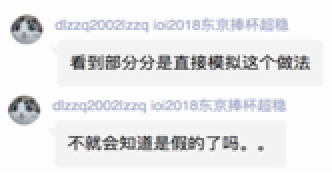# 博客

## UOJ Round #17 题解

2018-03-18 22:58:56 By WuHongxun

### 滑稽树上滑稽果

By jiry_2，数据、题解By WuHongxun懂我意思吧？

#### 子任务五dp转移就是每次枚举一个$a[i]$，$f[S] = min(f[S], f[S \& a[i]] + (S \& a[i]))$。

### 滑稽树下你和我

By picks，数据、题解By Scape

### 滑稽树前做游戏

By jiry_2

#### 算法二

$n = 4$ 的本质不同的图只有 $11$ 种，如果你有高超的手算水平，相信你可以算出所有 $n \leq 4$ 的图的答案，然后打表。

#### 算法三

1. 如果所有点的权值都小于等于 $\frac{t}{2}$，那么必定满足顶点权值最大值小于等于 $y$，因此此时的概率为 $(\frac{t}{2})^n$（令 $G$ 的顶点个数为 $n$）。

2. 如果至少有一个点的权值大于 $\frac{t}{2}$，我们枚举权值最大的点 $i$，设它的权值为 $w$。考虑所有与 $i$ 相邻的点 $j$，所有 $j$ 的出边中，权值最大的就是边 $(i,j)$ 了，因此 $j$ 的权值应当小于等于 $t-w$（又 $w>\frac{t}{2}$，因此不会和其他限制导出矛盾）。我们删去第 $i$ 个点和所有相邻点，得到了图 $G_i$，那么 $G_i$ 中的点应当满足点权小于等于 $w$ 且答案不超过 $t$，即概率为 $h(G_i,w,t)$。

$$h(G,y,t) = (\frac{t}{2})^n + \sum_{i=1}^n \int_{\frac{t}{2}}^{y}h(G_i,w,t)\text{d}w$$

oscar

diamond_duke

Trisolaris